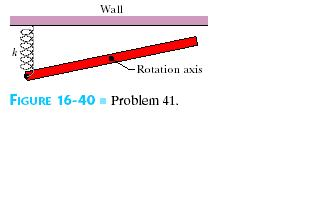Ask question

# A triangle is rotated 180 about the origin. Its image is reflected in the x-axis. The verticles of the final triangle are (-4,-4),(-2,-4), and (-3,-1). That are the verticles of the original triangle?# A triangle is rotated 180 about the origin. Its image is reflected in the x-axis. The verticles of the final triangle are (-4,-4),(-2,-4), and (-3,-1). That are the verticles of the original triangle?

Question
Right triangles and trigonometryasked 2021-03-01
A triangle is rotated 180 about the origin. Its image is reflected in the x-axis. The verticles of the final triangle are (-4,-4),(-2,-4), and (-3,-1). That are the verticles of the original triangle?

## Answers (1)2021-03-02
Before being reflected in the xx-axis the vertices (x,y) of the triangle were:
$$\displaystyle{\left({x},{y}\right)}→{\left({x},−{y}\right)}$$
(−4,4), (−2,4), (−3,1),
Before being rotated 180\textdegree180\textdegree about the origin, the vertices (x,y) of the triangle were:
$$\displaystyle{\left({x},{y}\right)}→{\left(−{x},−{y}\right)}$$
(4,−4). (2,−4). (3,−1).

### Relevant Questionsasked 2021-02-14
Mg reacts with H+ (aq) according to
$$\displaystyle{M}{g{{\left({s}\right)}}}+{2}{H}+{\left({a}{q}\right)}\to{M}{g}_{{2}}+{\left({a}{q}\right)}+{H}_{{2}}{\left({g}\right)}$$
Suppose that 0.524 g of Mg is reacted with 60.o ml of 1.0 M H+(aq). Assume that the density of the H+ (aq) solution os 1.00 g/ml,and that its specific heat capacity equals that of water. Theinitial and final temperatures are 22.0 degree celsius and 65.8degree celsius.
a) Is the reaction endothermic or exothermic?
b) Calculate $$\displaystyle\triangle{H}$$ of of th reaction. Use correct sigs andgive units.
c) Calculate the $$\displaystyle\triangle{H}$$ of the reaction per mole of magnesium.asked 2021-05-04
Assume that a 1.00-kg ball is thrown solely by the action of the forearm, which rotates about the elbow joint under the action of the triceps muscle. The ball is accelerated uniformly from rest to 10.0 m/s in 0.350 s, at which point it is released. Calculate (a) the angular acceleration of the arm, and (b) the force required of the triceps muscle. Assume that the forearm has a mass of 3.70 kg and rotates like a uniform rod about an axis at its end.asked 2021-05-08
A high-speed sander has a disk 4.00 cm in radius that rotates about its axis at aconstant rate of 1265 rev/min.Determine
(a) the angular speed of the disk in radians persecond,
rad/s
(b) the linear speed of a point 2.2 cmfrom the disk's center,
m/s
(c) the centripetal acceleration of a point on the rim, and
$$\displaystyle\frac{{m}}{{s}^{{{2}}}}$$
(d) the total distance traveled by a point on the rim in1.96 s.
masked 2021-05-10
In the overhead view of, a long uniform rod of mass m0.6 Kg is free to rotate in a horizontal planeabout a vertical axis through its center .A spring with force constant k = 1850 N/m is connected horizontally betweenone end of the rod and a fixed wall. When the rod is in equilibrium, it is parallel to the wall. What isthe period of the small oscillations thatresult when the rod is rotated slightly and released?asked 2021-04-26
A thin piece if wire 4.00mm long is located in a plpaneperpendicular to the optical axis and 60.0cm in front of a thinlens. The sharp image of the wire formed on a screen is 2.0mm long.What is the focal length of the lens?asked 2021-04-04
Water at a pressure of $$\displaystyle{3.00}\times{10}^{{5}}$$ Pa flows through a horizontal pipe at a speed of 1.00 m/s. the pipe narrows to 1/4 its original diameter. Find the following:
A. The flow speed in the narrow section
B. the pressure in the narrow sectionasked 2021-04-25
A log 10 m long is cut at 1 meter intervals and itscross-sectional areas A (at a distance x from theend of the log) are listed in the table. Use the Midpoint Rule withn = 5 to estimate the volume of the log. (in $$\displaystyle{m}^{{{3}}}$$ )Show transcribed image text A log 10 m long is cut at 1 meter intervals and itscross-sectional areas A (at a distance x from theend of the log) are listed in the table. Use the Midpoint Rule withn = 5 to estimate the volume of the log. (in $$\displaystyle{m}^{{{3}}}$$)asked 2021-03-12
Aidan knows that the observation deck on the Vancouver Lookout is 130 m above the ground. He measures the angle between the ground and his line of sight to the observation deck as $$\displaystyle{77}^{\circ}$$. How far is Aidan from the base of the Lookout to the nearest metre?asked 2021-05-12
4.7 A multiprocessor with eight processors has 20attached tape drives. There is a large number of jobs submitted tothe system that each require a maximum of four tape drives tocomplete execution. Assume that each job starts running with onlythree tape drives for a long period before requiring the fourthtape drive for a short period toward the end of its operation. Alsoassume an endless supply of such jobs.
a) Assume the scheduler in the OS will not start a job unlessthere are four tape drives available. When a job is started, fourdrives are assigned immediately and are not released until the jobfinishes. What is the maximum number of jobs that can be inprogress at once? What is the maximum and minimum number of tapedrives that may be left idle as a result of this policy?
b) Suggest an alternative policy to improve tape driveutilization and at the same time avoid system deadlock. What is themaximum number of jobs that can be in progress at once? What arethe bounds on the number of idling tape drives?asked 2021-05-10
Hypothetical potential energy curve for aparticle of mass m
If the particle is released from rest at position r0, its speed atposition 2r0, is most nearly
a) $$\displaystyle{\left({\frac{{{8}{U}{o}}}{{{m}}}}\right)}^{{1}}{\left\lbrace/{2}\right\rbrace}$$
b) $$\displaystyle{\left({\frac{{{6}{U}{o}}}{{{m}}}}\right)}^{{\frac{{1}}{{2}}}}$$
c) $$\displaystyle{\left({\frac{{{4}{U}{o}}}{{{m}}}}\right)}^{{\frac{{1}}{{2}}}}$$
d) $$\displaystyle{\left({\frac{{{2}{U}{o}}}{{{m}}}}\right)}^{{\frac{{1}}{{2}}}}$$
e) $$\displaystyle{\left({\frac{{{U}{o}}}{{{m}}}}\right)}^{{\frac{{1}}{{2}}}}$$
if the potential energy function is given by
$$\displaystyle{U}{\left({r}\right)}={b}{r}^{{P}}-\frac{{3}}{{2}}\rbrace+{c}$$
where b and c are constants
which of the following is an edxpression of the force on theparticle?
1) $$\displaystyle{\frac{{{3}{b}}}{{{2}}}}{\left({r}^{{-\frac{{5}}{{2}}}}\right)}$$
2) $$\displaystyle{\frac{{{3}{b}}}{{{2}}}}{\left\lbrace{3}{b}\right\rbrace}{\left\lbrace{2}\right\rbrace}{\left({r}^{{-\frac{{1}}{{2}}}}\right)}$$
3) $$\displaystyle{\frac{{{3}{b}}}{{{2}}}}{\left\lbrace{3}\right\rbrace}{\left\lbrace{2}\right\rbrace}{\left({r}^{{-\frac{{1}}{{2}}}}\right)}$$
4) $$\displaystyle{2}{b}{\left({r}^{{-\frac{{1}}{{2}}}}\right)}+{c}{r}$$
5) $$\displaystyle{\frac{{{3}{b}}}{{{2}}}}{\left\lbrace{2}{b}\right\rbrace}{\left\lbrace{5}\right\rbrace}{\left({r}^{{-\frac{{5}}{{2}}}}\right)}+{c}{r}$$
...